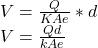## A parallel-plate capacitor has the volume between its plates filled with plastic with dielectric constant K. The magnitude of the charge on

Question

A parallel-plate capacitor has the volume between its plates filled with plastic with dielectric constant K. The magnitude of the charge on each plate is Q. Each plate has area A, and the distance between the plates is d.1. Use Gauss’s law to calculate the magnitude of the electric field in the dielectric.2. Express your answer in terms of the given quantities and appropriate constants.3. Use the electric field determined in part A to calculate the potential difference between the two plates.4. Express your answer in terms of the given quantities and appropriate constants.Use the result of part B to determine the capacitance of the capacitor.5. Express your answer in terms of the given quantities and appropriate constants.

in progress 0
3 weeks 2021-08-30T16:20:50+00:00 1 Answers 0 views 0

a) E =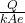b)V =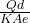Explanation:

Thinking process:

Given that:

magnitude of charge on each plate  = Q

Area of each plate = A

Dielectric constant = K

Distance between the plate = d

Let the area of the enclosed charge A be: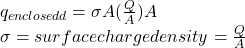According to Gauss’ Law: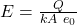b) The relation between the potential d:

V = Ed

where d = distance between the plate

Plugging in the value of E: